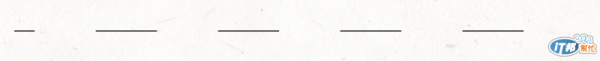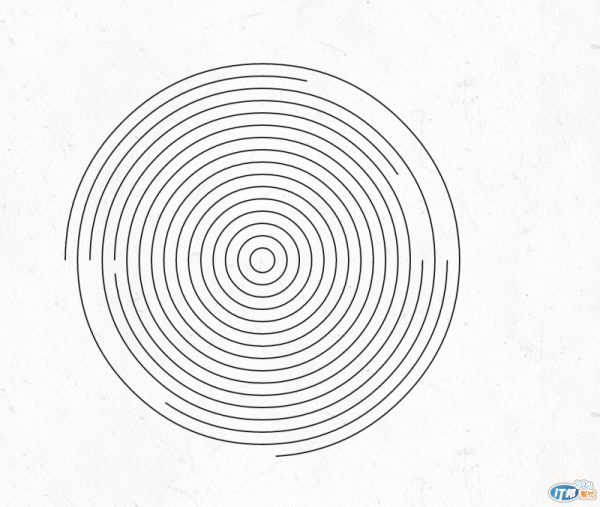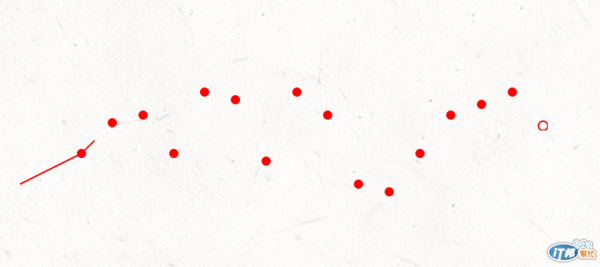#DAY 6
2

## Stroke Dash

http://cssdeck.com/labs/ironman-svg-line-animation

stroke-dasharray + stroke-dashoffset

stroke-dasharray是把stroke做成了是虛線的效果，線段會被拆成線段、空白、線段、空白，效果就像下面這樣。

line {
stroke-dasharray: 60;
}stroke-dashoffset

• 推移後，dasharray還是會保持循環。
• dashoffset的值不會大於dasharray。
• 如果dashoffset 等於 dasharray，線段起始點會是空白。

line.l2{
stroke-dashoffset: 40;
}line.l3{
stroke-dasharray: 200;
stroke-dashoffset: 200;
}## 結合CSS aniamtion

aniamtion 相關的瀏覽器前輟詞問題，可參考/css/2013/10/17/css-animation-keyframe/

//sass
.stroke
stroke-dashoffset: 2000
stroke-dasharray: 2000

//動畫效果 10秒 線性動畫 無限循環
.ani
animation: circle-draw 10s linear infinite

//keyframes的值
//將dashoffset拉回至0
@keyframes circle-draw
80%
stroke-dashoffset: 0<svg class="stroke ani" height="400" width="100%" >
<g>
<circle cx="200" cy="200" r="10"/>
<circle cx="200" cy="200" r="20"/>
<circle cx="200" cy="200" r="30"/>
<circle cx="200" cy="200" r="40"/>
<circle cx="200" cy="200" r="50"/>
<circle cx="200" cy="200" r="60"/>
<circle cx="200" cy="200" r="70"/>
<circle cx="200" cy="200" r="80"/>
<circle cx="200" cy="200" r="90"/>
<circle cx="200" cy="200" r="100"/>
<circle cx="200" cy="200" r="110"/>
<circle cx="200" cy="200" r="120"/>
<circle cx="200" cy="200" r="130"/>
<circle cx="200" cy="200" r="140"/>
<circle cx="200" cy="200" r="150"/>
<circle cx="200" cy="200" r="160"/>
</g>
</svg>

## 回到Marker

<svg width="100%" height="200">
<defs>
<marker id="midCircle" markerWidth="8" markerHeight="8" refx="5" refy="5">
<circle id="dot" cx="5" cy="5" r="3" fill="red" />
</marker>
<marker id="endCircle" markerWidth="8" markerHeight="8" refx="5" refy="5">
<circle id="dot" cx="5" cy="5" r="3" fill="white" stroke="red" />
</marker>
</defs>
<polyline class="stroke ani" points="20,140 60,120 80,100 100,95 120,120 140,80 160,85 180,125 200,80 220,95 240,140 260,145 280,120 300,95 320,88 340,80 360,102"
style="fill:none;
stroke:red;
stroke-width:1;
marker-mid: url(#midCircle);
marker-end: url(#endCircle);
" />
</svg>SVG 與 D3.js30